# Work, Power & Energy- JEE Advanced Previous Year Questions with Solutions

JEE Advanced Previous Year Questions of Physics with Solutions are available at eSaral. Practicing JEE Advanced Previous Year Papers Questions of Physics will help the JEE aspirants in realizing the question pattern as well as help in analyzing weak & strong areas. Get detailed Class 11th & 12th Physics Notes to prepare for Boards as well as competitive exams like IIT JEE, NEET etc. eSaral helps the students in clearing and understanding each topic in a better way. eSaral is providing complete chapter-wise notes of Class 11th and 12th both for all subjects.       Download eSaral app  for free study material and video tutorials.   Simulator Previous Years JEE Advanced Questions
Q. A light inextensible string that goes over a smooth fixed pulley as shown in the figure connects two blocks of masses 0.36 kg and 0.72 kg. Taking g = 10 $\mathrm{m} / \mathrm{s}^{2}$, find the work done (in joules) by the string on the block of mass 0.36 kg during the first second after the system is released from rest.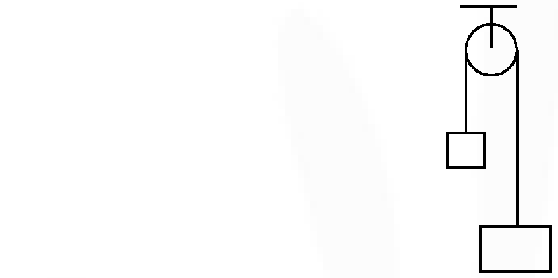[IIT-JEE-2009]
Ans. 8 $\mathrm{a}=\frac{(0.73-0.36) \times 10}{(0.72+0.36)}$ $=\frac{10}{3} \mathrm{m} / \mathrm{s}^{2}$ $\mathrm{T}=0.36\left(10+\frac{10}{3}\right)=4.8 \mathrm{N}$ $\Delta y=\frac{1}{2} \times \frac{10}{3} \times 1^{2}$ $\mathrm{W}_{\mathrm{T}}=4.8 \times \frac{5}{3}=8 \mathrm{J}$
Q. A light inextensible string that goes over a smooth fixed pulley as shown in the figure connects two blocks of masses 0.36 kg and 0.72 kg. Taking g = 10 m/s2, find the work done (in joules) by the string on the block of mass 0.36 kg during the first second after the system is released from rest.[IIT-JEE-2009]
Ans. $-\frac{1}{2} \times 2 \times(0.06)^{2}-0.18 \times 0.06=-\frac{1}{2} \times 0.18 \times v^{2}$ $\Rightarrow 0.0036+0.0108=0.09 \mathrm{v}^{2}$ $\Rightarrow \mathrm{v}=0.4=\frac{4}{10} \mathrm{m} / \mathrm{sec}$
Q. A block of mass 0.18 kg is attached to a spring of force-constant 2 N/m. The coefficient of friction between the block and the floor is 0.1. Initially the block is at rest and the spring is un-stretched. An impulse is given to the block as shown in the figure. The block slides a distance of 0.06 m and comes to rest for the first time. The initial velocity of the block in m/s is V= N/10. Then N is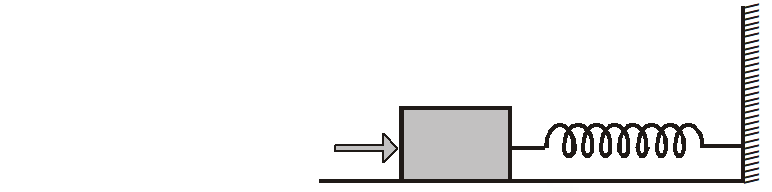[IIT-JEE-2011]
Ans. 0 $\overrightarrow{\mathrm{F}}=\mathrm{k}\left[\frac{\overrightarrow{\mathrm{r}}}{\mathrm{r}^{3}}\right] \Rightarrow$ force is radial. So W = 0
Q. A particle of mass 0.2 kg is moving in one dimension under a force that delivers a constant power 0.5 W to the particle. If the initial speed $\left(\text { in } \mathrm{ms}^{-1}\right)$ of the particle is zero, the speed $\left(\text { in } \mathrm{ms}^{-1}\right)$ after 5 s is. [JEE-Advance-2013]
Ans. 5t $\mathrm{P}=\mathrm{F} \cdot \mathrm{v} \Rightarrow 0.5=0.2 \mathrm{v} \frac{\mathrm{dv}}{\mathrm{dt}} \Rightarrow \mathrm{v}^{2}=5 \mathrm{t}$
Paragraph for Questions 5 and 6 A small block of mass 1 kg is released from rest at the top of a rough track. The track is a circular arc of radius 40 m. The block slides along the track without toppling and a frictional force acts on it in the direction opposite to the instantaneous velocity. The work done in overcoming the friction up to the point Q, as shown in the figure below, is 150 J. (Take the acceleration due to gravity, g = 10 $\mathrm{m} \mathrm{s}^{-2}$)
Q. The magnitude of the normal reaction that acts on the block at the point Q is (A) 7.5 N (B) 8.6 N (C) 11.5 N (D) 22.5 N [JEE-Advance-2013]
Ans. (A)
Q. The speed of the block when it reaches the point Q is (A) $5 \mathrm{ms}^{-1}$ (B) $10 \mathrm{ms}^{-1}$ (C) $10 \sqrt{3} \mathrm{ms}^{-1}$ (D) $20 \mathrm{ms}^{-1}$ [JEE-Advance-2013]
Ans. (B)
Q. Consider an elliptically shaped rail PQ in the vertical plane with OP = 3 m and OQ = 4m. A block of mass 1 kg is pulled along the rail from P to Q with a force of 18 N, which is always parallel to line PQ (see the figure given). Assuming no frictional losses, the kinetic energy of the block when it reaches Q is (n × 10) Joules. The value of n is (take acceleration due to gravity = 10 $\mathrm{ms}^{-2}$ )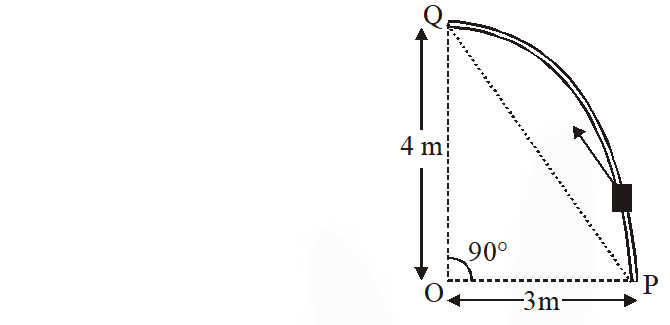[JEE-Advance-2014]
Ans. 5 $\mathrm{K}_{\mathrm{f}}-\mathrm{K}_{\mathrm{i}}=\mathrm{W}_{\mathrm{all}}$ $\mathrm{K}_{\mathrm{f}}=\mathrm{W}_{\mathrm{ext}}+\mathrm{W}_{\mathrm{gr}}$ = 18 × 5 J – 1 × 10 × 4 J = 50 J = 5 × 10 J
Q. A wire, which passes through the hole in a small bead, is bent in the form of quarter of a circle. The wire is fixed vertically on ground as shown in the figure. The bead is released from near the top of the wire and it slides along the wire without friction. As the bead moves from A to B, the force it applies on the wire is :-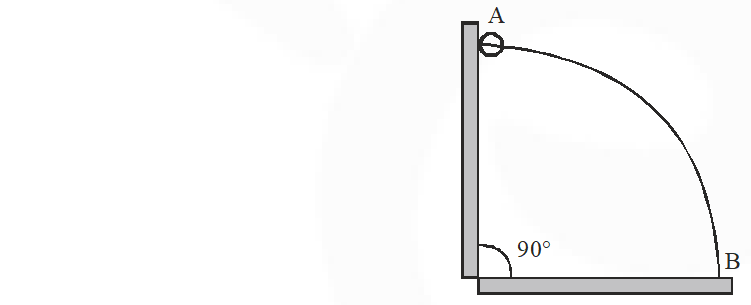(A) Always radially outwards (B) Always radially inwards (C) Radially outwards initially and radially inwards later. (D) Radially inwards initially and radially outwards later. [JEE Advanced-2014]
Ans. (D)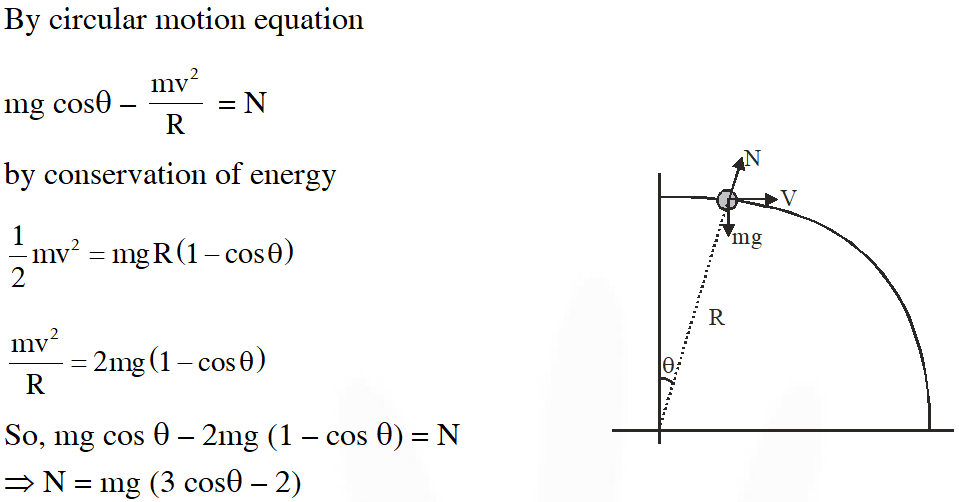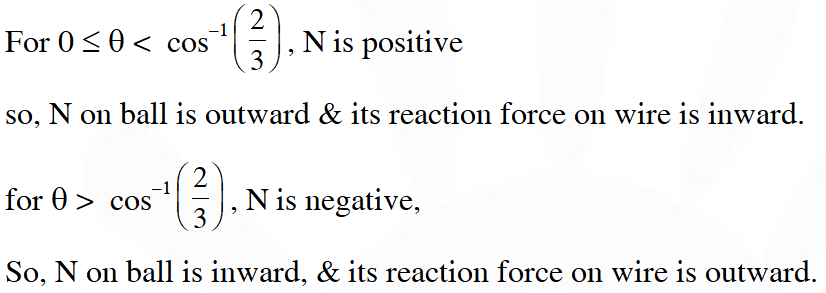Q. A particle of mass m is initially at rest at the origin. It is subjected to a force and starts moving along the x-axis. Its kinetic energy K changes with time as dK/dt = $\gamma \mathrm{t}$, where  is a positive constant of appropriate dimensions. Which of the following statements is (are) true ? (A) The force applied on the particle is constant (B) The speed of the particle is proportional to time (C) The distance of the particle from the origin increses linerarly with time (D) The force is conservative [JEE Advanced-2018]
Ans. (A,B,D) $\frac{\mathrm{d} \mathrm{k}}{\mathrm{dt}}=\gamma \mathrm{t}$ as $\mathrm{k}=\frac{1}{2} \mathrm{mv}^{2}$ $\therefore \frac{\mathrm{dk}}{\mathrm{dt}}=\mathrm{mv} \frac{\mathrm{d} \mathrm{v}}{\mathrm{dt}}=\gamma \mathrm{t}$ $\therefore \mathrm{m} \int_{0}^{\mathrm{v}} \mathrm{vdv}=\gamma \int_{0}^{\mathrm{t}} \mathrm{tdt}$ $\frac{\mathrm{mv}^{2}}{2}=\frac{\gamma \mathrm{t}^{2}}{2}$ $\mathrm{v}=\sqrt{\frac{\gamma}{\mathrm{m}} \mathrm{t}} \textrm{ } \quad \ldots \ldots$ (i) $\mathrm{a}=\frac{\mathrm{d} \mathrm{v}}{\mathrm{dt}}=\sqrt{\frac{\gamma}{\mathrm{m}}}=\mathrm{constant}$ since F = ma $\therefore \mathrm{F}=\mathrm{m} \sqrt{\frac{\gamma}{\mathrm{m}}}=\sqrt{\gamma \mathrm{m}}=\mathrm{constant}$

Albertojaige
Feb. 25, 2023, 7:07 p.m.
Nicely put. Kudos!
anurag
May 5, 2022, 8:32 p.m.
b
Sept. 30, 2021, 2 p.m.
scam
sdas
June 19, 2021, 12:29 a.m.
love
Feb. 24, 2021, 1:01 p.m.
Yashasvi Arya
Nov. 17, 2020, 6:18 a.m.
Ye bhi theek h !!
Binod Tharu
Oct. 5, 2020, 11:34 a.m.
The questions are too less
Srikar
Oct. 4, 2020, 7:45 p.m.
👍
Sritam Sriyanshu Nanda
Oct. 4, 2020, 9:30 a.m.
Hehe
Srikar
Sept. 21, 2020, 9:17 p.m.
Super questions
hi
Sept. 19, 2020, 5:56 p.m.
thankyou
CHITTY
Sept. 17, 2020, 10:33 a.m.
IMPROVE NO.OF QUESTIONS
Yagyesh dubey
Sept. 13, 2020, 5:01 p.m.
Pleaee increase no. Of questions
keerthana
Sept. 11, 2020, 4:31 p.m.
very good collection. but there are less no of que
Apurba Das
Aug. 19, 2020, 12:36 a.m.
good questions but less in numbers
munna bhai
Aug. 2, 2020, 8:57 a.m.
less no.of questions
Abc
Aug. 1, 2020, 11:17 p.m.
Tq
NARENDAR Banoth
July 27, 2020, 8:19 p.m.